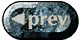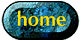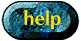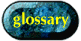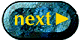How to build a phase diagram page 1 of 6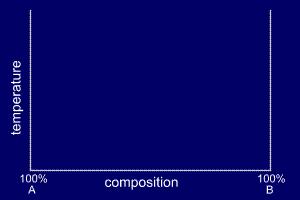A binary phase diagram shows the phases formed in differing mixtures of two elements over a range of temperatures.Compositions run from 100% Element A on the left of the diagram, through all possible mixtures, to 100% Element B on the right. The composition of an alloy is given in the form A - x%B. For example, Cu - 20%Al is 80% copper and 20% aluminium. Weight percentages are often used to specify the proportions of the alloying elements, but atomic percent may be used. The type of percentage is specified e.g. Cu - 20wt%Al for weight percentages and Cu - 20at%Al for atomic percentages. Weight percentages will be used throughout this text.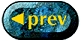How to build a phase diagram page 2 of 6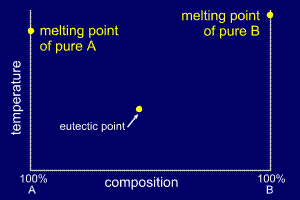Alloys tend to solidify over a temperature range, rather than at a specific temperature like pure elements.At each end of the phase diagram only one of the elements is present (100% A or 100% B) and therefore a specific melting point exists. Sometimes there is a mixture of the constituent elements which produces solidification at a single temperature like a pure element. This is called the eutectic point. The eutectic point can be found experimentally by plotting cooling rates over ranges of alloy composition. The phase diagrams for some very simple binary alloys do not have eutectic points (more info).How to build a phase diagram page 3 of 6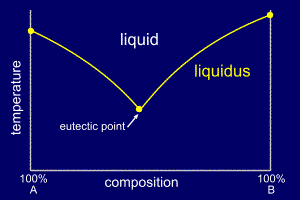By cooling alloys from the liquid state and recording their cooling rates, the temperature at which they start to solidify can be determined and then plotted on the phase diagram. If enough experiments are performed over a range of compositions, a start of solidification curve can be plotted onto the phase diagram.This curve will join the three single solidification points and is called the liquidus line.How to build a phase diagram page 4 of 6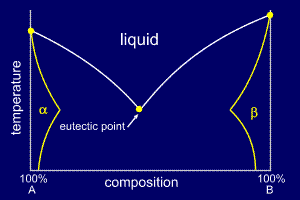In the same way that sugar dissolves into hot tea (a liquid solution) it is possible for one element to dissolve in another, whist both remain inn the solid state. This is called solid solubility and is characteristically up to a few percent by weight. This solubility limit will normally change with temperature.The extent of the solid solubility region can be plotted onto the phase diagram and labelled appropriately. A solid solution of B in A (i.e. mostly A) is called alpha and a solid solution of A in B (i.e. mostly B) is called beta. It is worthwhile to note that some elements that are alloyed have zero solid solubility; a good example is Al - Si alloys, where aluminium has zero solid solubility in silicon.How to build a phase diagram page 5 of 6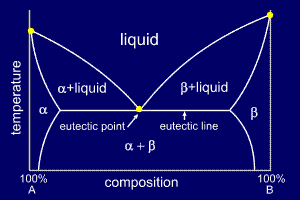If an alloy's composition does not place it within the small solid solution regions at either side of the phase diagram, the alloy will become fully solid at the eutectic temperature, shown as the eutectic line on the phase diagram.At alloy compositions and temperatures between the start of solidification and the point at which it becomes fully solid (the eutectic temperature) a mushy mix of either alpha or beta will exist as solid lumps with a liquid mixture of A and B. These partially solid regions are marked on the phase diagram. The region below the eutectic line, and outside the solid solution region, will be a solid mixture of alpha and beta, and is labelled to reflect this.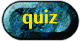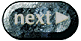How to build a phase diagram page 6 of 6A phase diagram is a temperature - composition map which indicates the phases present at a given temperature and composition.It is determined experimentally by recording cooling rates over a range of compositions. We will use these diagrams to understand and predict the alloy microstructure obtained at a given composition.A simple phase diagram more info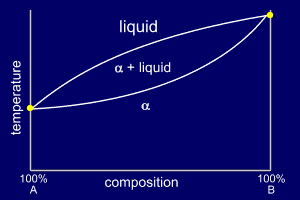Very simple binary phase diagrams do not have a eutectic point. The liquid mixture will cool through a solidification region (temperature range) and become a solid solution of the two constituent elements.These simple phase diagrams normally only occur when two very similar elements are being alloyed or as part of a more complex phase diagram.